# Gravitation, theory of

A branch of field theory in theoretical and mathematical physics, extensively using geometrical methods of investigation. The traditional subject of the theory of gravitation is the study of gravitational interaction between material objects, acting on their movement and structure (cf. Gravitation). The subject of the theory of gravitation embraces, besides the analysis of the gravitational field itself, also the space-time structure more generally, the problem of quantization of gravity and its relations to the theory of elementary particles. Correspondingly, the mathematical apparatus used in the theory of gravitation extended from the theory of second-order ordinary and partial differential equations to differential (pseudo-Riemannian) geometry, to the theory of functions of several complex variables and complex manifolds, to topology, to the theory of groups, as well as to spinor and twistor calculus. More and more often computers are being used to do calculations (including symbolic calculations).

The foundations of the theory of gravitation were laid from the end of the 16th century onwards until the beginning of the 18th century in the works of G. Galilei and I. Newton. In Newton's classical theory of gravitation the equation for the potentialof the gravitational field has the form of the Poisson equation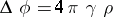whereis Newton's gravitational constant andis the mass density of the field sources. The field strength is defined as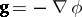, while the force with which the field acts on a given test point massis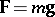(the test mass itself does not disturb the field). Newton's second law then gives the equation of motion of a test mass. In a concrete setting, Newton's theory of gravitation is applied to a number of problems in, e.g., ballistics and celestial mechanics. Up till now it is sufficiently exact for the description of practically all of celestial mechanics. As a theory based on potentials, Newton's theory of gravitation was (subsequently) a model for constructing the theory of electrostatics, while the notion of a physical field, formulated in Maxwell's electrodynamics, has had in turn an influence on the birth of Einstein's relativistic theory of gravitation.

A. Einstein started the construction of a new theory of gravitation with the introduction of the principle of finiteness of the velocity of propagation of interactions (including the gravitational one) and of the equivalence principle. He made the first step in this direction in 1907 and defined, in an article together with M. Grossmann (1913), the way to the construction of a relativistic theory of gravitation (general relativity theory) as geometrization of physics. The idea that a non-Euclidean geometry could be the actual one was coined by C.F. Gauss and N.I. Lobachevskii. In a sufficiently outlined form it was expounded by B. Riemann and W. Clifford; however, it was Einstein who first related gravity with the geometry not of three-dimensional space but with that of four-dimensional space-time, which was to play a decisive role. The gravitational field equations were given in a final form by D. Hilbert (1915) and Einstein (1916, who correctly stated them earlier for a field in vacuum).

In the relativistic theory of gravitation the geometrical characteristics of the space-time manifold play at the same time the role of variables describing the gravitational field. In the squared line element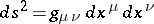, which metrizes space-time, the indefinite metric tensor (here of signature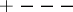) plays the role of the multi-component gravitational potential. The equation of the light cone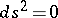is used in the formulation of the general-relativistic causality principle. The connection coefficients, determining parallel displacement and covariant differentiation (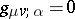), play the role of field strengths. The Riemann curvature tensor (cf. Riemann tensor) can be expressed as a combination of derivatives of those field strengths, i.e. characterizes the non-homogeneity of this field. The Ricci tensor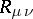(the contracted curvature tensor, the "divergence of the field strengths" in classical potential theory) can be algebraically expressed in terms of the energy-momentum (stress-energy) tensor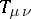of the gravitational field sources, i.e. of matter and fields (except the gravitational field itself), thus giving the Einstein equations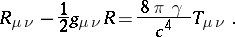(1)

The left-hand side of these equations is non-linear in the metric tensor and its first derivatives, but in the case of a weak gravitational field the non-linearity may be separated as an individual tensor which, after being carried over to the right-hand side, can be combined with the sources (hence the treatment of the non-linearity of a gravitational field as an expression of its self-interaction). The equation of motion of a test point mass in an external gravitational field can be written as the equation of geodesics(2)

and does not contain the mass of the particle (i.e. under otherwise identical conditions, test point particles of various masses move identically). This expresses the equivalence principle, corresponding here to the equality of the inertial and the gravitational masses (a fact that was experimentally confirmed with a precision of; this work was done by R. Eötvös, R. Dicke; the precision mentioned was obtained by V.B. Braginskii). In another formulation, known since Einstein's first work, this principle states the local equivalence of gravitation and acceleration: In a freely-falling laboratory without rotation, occupying a small space, and for a short time, one can not notice the existence of gravitation, whatever physical experiment one performs. Equation (2) is the first approximation to the problem for systems of non-test bodies. In 1938–1939 Einstein, L. Infeld, B. Hoffmann and V.A. Fock [V.A. Fok] discovered simultaneously a method for finding the further approximations (the-body problem in general relativity theory). The equations of other (non-gravitational) fields, e.g. an electromagnetic field, are obtained from the usual theory of the corresponding fields in the flat world under the condition of general covariance. All field equations, including (1), as well as the equation of motion (2), follow from the principle of extremal action with correspondingly given Lagrange functions. In the general theory one examines self-consistency of systems of equations, but often, in view of their complexity, some or all non-gravitational fields are treated as test fields (for them a non-self-consistent problem with external gravitational fields is to be solved).

Because of the non-linearity of Einstein's equations special methods for solving them exactly have been developed (approximate solutions can not reflect the specifics of a problem). The choice of a suitable basis (tetrad) is specially important. Cartan's method of exterior forms is often used (cf. Cartan method of exterior forms). A complex null (light-like) tetrad is used in the very effective Newman–Penrose formalism (see ). As applied to Einstein's equations, new methods of Båcklund-transformation type of the inverse scattering problem have been developed (the theory of solitons, cf. Soliton). Already, a large number of exact solutions to the Einstein equations are known (cf. ). The first of which (the Schwarzschild solution of 1916,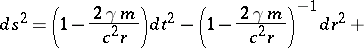(3)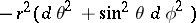in the commonly-used curvilinear coordinate system) has the meaning of the gravitational field of a spherically-symmetric point mass. From the physical point of view, solutions to Einstein's equations may be subdivided into vacuum fields outside local sources, gravitational fields of matter distributions and other fields, Einstein–Maxwell fields, gravitational wave fields, cosmological solutions, etc. Various methods for classifying the pseudo-Riemannian spaces that aid in constructing solutions to Einstein's equations with desired properties and in interpreting already-known solutions have been developed. These are: 1) an algebraic classification by properties of the Weyl conformal curvature tensor (the three Petrov types — I, II, III, and two degenerate subtypesand, as well as the trivial case, type 0, corresponding to conformally-flat spaces; it is often taken thatand III correspond to gravitational wave fields); 2) an algebraic classification by properties of the Ricci tensor (or energy-momentum tensor); and 3) a classification by groups of motions (isometries: mappings of a space-time onto itself by Lie displacements without changing the metric). In-dimensional space, supposing it is homogeneous, the last classification leads to the nine Bianchi types, which play an important role in the theory of homogeneous cosmological models. To obtain, and especially, to study solutions of Einstein's equations one more and more often uses a computer; symbolic computations are successfully employed in this area. There are reports that the identification problem for metrics given in differential coordinate systems has been solved with the aid of a computer. To compare the inferences from Einstein's theory of gravitation and its various modifications and generalizations there has been an attempt to develop a phenomenological method of description of the metric tensor and gravitational effects ( "parametrized post-Newtonian theory of gravitation" ).

When analyzing concrete solutions of Einstein's equations, an important role is played by the problem of the completeness of the atlas of charts of the given manifold (hence the development of extension methods); the search and investigation of singularities (their definition is made fundamentally more difficult by the indefiniteness of the metric); and the computation of asymptotic (including topological) properties of solutions in the case of insular systems. Problems in Einstein's theory of gravitation lead to the formulation of a new important concept in pseudo-Riemannian geometry — that of a horizon. Although a horizon (one distinguishes between the event, the particles, the Cauchy, the causality, and the apparent horizon) is not a set of singular points, it can be invariantly distinguished in space-time. Thus, the event horizon in an asymptotically-flat world is defined as the limit of the set of events, i.e. of-dimensional points, from which one still may leave towards infinity while remaining within the light cone. If an event horizon exists, then the domain bounded by it in space-time (from which it is impossible to go to infinity without crossing the light cone) is called the black hole; the simplest example of it is described by the extension of the Schwarzschild metric (3). Inside the black hole there are singularities (in particular, some invariants of the Riemann curvature of (3) diverge (go to infinity) as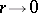). Moreover, the Schwarzschild singularity is space-like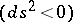, while for other black holes (the general case is a Kerr–Newman metric, ) it may also be time-like (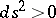). Astrophysical applications of general relativity theory have shown that black holes can be formed as result of gravitational collapse of massive stars; a series of candidates for the role of black hole have been considered among the observed celestial bodies which could be disclosed by processes occurring in their outer domains where the gravitational forces are strong. It is considered that under so-called energy conditions (in fact: natural conditions on the energy-momentum tensor of matter) a singular state in the past and future under the evolution of material systems is inevitable (the singularity theorems of R. Penrose, S. Hawking, et al.). However, it is conjectured that the singularities are hidden by the horizons (the "cosmic censorship" hypothesis).

The foundations of the quantum theory of gravitation (both in the sense of quantizing a gravitational field and of quantizing other fields on the background of non-flat classical geometries) have been developed. One of the consequences is Hawking's effect of particle (photon, etc.) generation by black holes, leading to their "evaporation" . Quantum effects of gravitation are important at the early stages of the expansion of the Universe. In the description of the quantum theory of gravitation one uses on the canonical formalism of field theory, Feynman path integrals, etc.; related to this one has developed Euclidean field theory (in the sense of signature), has investigated instanton solutions to Einstein's equations, and has developed the Penrose twistor calculus, which is in its results close to the theory of complexified spaces with a self-dual or anti-self-dual Weyl conformal curvature tensor (-spaces of E. Newman). Other generalizations of the theory of gravitation include: the Einstein–Cartan theory with curvature and torsion; the affine theory of gravitation; the theory of gravitation with Lagrangian quadratic in the curvature; the bimetric theories of gravitation, etc. (cf. ).

Einstein's theory of gravitation leads to effects that are new as compared with Newton's theory; however, these effects are difficult to discover experimentally. Apart from these, both theories are in mutual agreement. Up till now, the following effects have been verified: gravitational red shift; bending of light rays; perihelion advance of a planetary orbit; non-stationarity (expansion) of the Universe. The effects of gravitational radiation have been verified indirectly (by the loss of energy of a two-body system revolving around a common centre of mass). Not a single fact contradicting Einstein's theory of gravitation has been found. On the edge of the reach of experiments today are: gravitational waves coming from cosmic sources, and dragging effects in gravitational fields on rotating bodies (precession of the axis of a gyroscope and others).